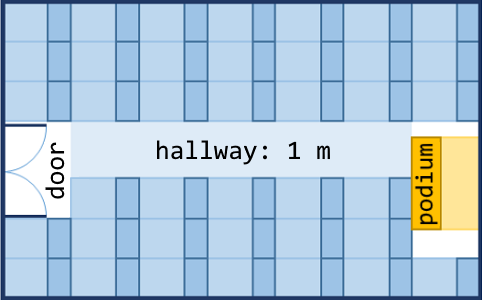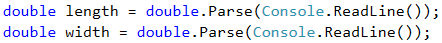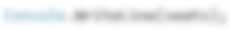# Problem: Training Lab

A training lab has a rectangular size l x w meters, without columns on the inside. The hall is divided into two parts- left and right, with a hallway approximately in the middle. In both parts, there are rows with desks. In the back of the hall, there is a big entrance door. In the front, there is a podium for the lecturer. A single working place takes up 70 x 120 cm (a table with size 70 x 40 cm + space for a chair with size 70 x 80 cm). The hallway width is at least 100 cm. It is calculated that due to the entrance door (which has 160 cm opening), exactly one working space is lost, and due to the podium (which has size of 160 x 120 cm), exactly two working spaces are lost. Write a program that reads the size of the training lab as input parameters and calculates the number of working places in it (look at the figure).

## Input Data

Two numbers are read from the console, one per line: l (length in meters) and w (width in meters).

Constraints: 3 ≤ wl ≤ 100.

## Output Data

Print an integer: the number of working places in the training lab.

## Sample Input and Output

Input Output Figure
15
8.9
1298.4
5.2
39## Clarification of the Examples

In the first example, the hall length is 1500 cm. 12 rows can be situated in it (12 * 120 cm = 1440 + 60 cm difference). The hall width is 890 cm. 100 cm of them are for the hallway in the middle. The rest 790 cm can be situated by 11 desks per row (11 * 70 cm = 770 cm + 20 cm difference). Number of places = 12 * 11 - 3 = 132 - 3 = 129 (we have 12 rows with 11 working places = 132 minus 3 places for podium and entrance door).

In the second example, the hall length is 840 cm. 7 rows can be situated in it (7 * 120 cm = 840, no difference). The hall width is 520 cm. 100 cm from them are for the hallway in the middle. The rest 420 cm can be situated by 6 desks per row (6 * 70 cm = 420 cm, no difference). Number of places = 7 * 6 - 3 = 42 - 3 = 39 (we have 7 rows with 6 working places = 42 minus 3 places for podium and entrance door).

## Hints and Guidelines

Try to solve the problem on your own first. If you do not succeed, go through the hints.

### Idea for Solution

As with any programming task, it is important to build an idea for its solution, before having started to write code. Let's carefully go through the problem requirements. We have to write a program that calculates the number of working places in a training lab, where the number depends on the hall length and height. We notice that the provided input will be in meters and the information about how much space the working places and hallway take, will be in centimeters. To do the calculations, we will use the same measuring units, no matter whether we choose to convert length and height into centimeters or the other data in meters. The first option is used for the presented solution.

Next, we have to calculate how many columns and how many rows with desks will fit. We can calculate the columns by subtracting the width by the necessary space for the hallway (100 cm) and divide the difference by 70 cm (the length of a working place). We find the rows by dividing the length by 120 cm. Both operations can result in a real number with whole and fractional part, but we have to store only the whole part in a variable. In the end, we multiply the number of rows by the number of columns and divide it by 3 (the lost places for entrance door and podium). This is how we calculate the needed value.

### Choosing Data Types

From the example, we see that a real number with whole and fractional part can be given as an input, therefore, it is not appropriate to choose data type int. This is why we use double. Choosing data type for the next variables depends on the method we choose to solve the problem. As with any programming task, this one has more than one way to be solved. Two methods will be shown here.

### Solution – Variant I

It is time to go to the solution. We can divide it into three smaller tasks:

• Reading input from the console.
• Doing the calculations.
• Printing the output on the console.

The first thing we have to do is read the input from the console. With Console.ReadLine() we read the values from the console and with the function double.Parse(…) string is converted into double.Let's move to the calculations. The special part here is that after having divided the numbers, we have to store only the whole part of the result in a variable.Search in Google! Whenever we have an idea how to solve a particular problem, but we do not know how to write it in C# or we are dealing with one that many other people have had before us, the easiest way to solve it is by looking for information on the Internet.

In this case, we can try with the following search: "c# get whole number part of double". One possible way is to use the method Math.Truncate(…) as it works with double data types. For the number of rows and columns we create variables of the same type.### Solution – Variant II

Second method: As we already know, the operator for division / operates differently on integers and decimals. When dividing integer with integer, the result is also an integer. Therefore, we can search how to convert the real numbers that we have as values for the height and the width, into integers and then divide them.

In this case, there could be data loss after having removed the fractional part, so it is necessary that it is converted expressly (explicit typecasting). We use the operator for converting data (type) by replacing the word type with the needed data type and place it before the variable.With Console.WriteLine(…) we print the result on the console.## Testing in the Judge System

Test your solution here: https://judge.softuni.org/Contests/Practice/Index/505#0.